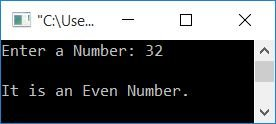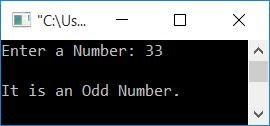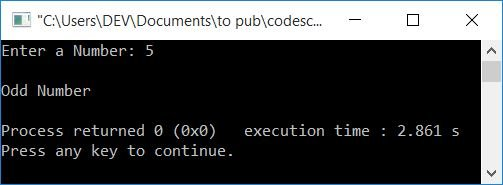# C++ program to check whether a given number is even or odd

In this article, you will learn how to check whether a given number is even or odd in C++ and get the code to do it. The program is created in the following two ways:

## Using if-else, determine whether the value is Even or Odd

To check whether the given number (by the user at run-time) is an even or an odd number in C++ programming, you have to ask the user to enter a number first. Now if it is divisible by 2 (without leaving any remainder), then it is an even number. Otherwise, it is an odd number.

```#include<iostream>
using namespace std;
int main()
{
int num;
cout<<"Enter a Number: ";
cin>>num;
if(num%2==0)
cout<<"\nIt is an Even Number.";
else
cout<<"\nIt is an Odd Number.";
cout<<endl;
return 0;
}```

This program was built and runs under the Code::Blocks IDE. Here is its sample run:Now enter any number to check whether it is an even or an odd number, as shown in the following output with user input as 32:Here is another sample run with user input as 33:With the user's input of 32, 32%2 gives 0. So the condition num%2==0 or 32%2==0 or 0==0 evaluates to true, therefore program flow goes inside the if's body and executes a statement that prints a message, "It is an Even Number.". In contrast, with a user input of 33, 33%2 yields 2. So the condition num%2==0 or 33%2==0 or 1==0 evaluates to false; therefore, in this case, program flow goes to else's body and executes a statement available in the body of else that prints a message, "It is an Odd Number."

## Using the Ternary Operator, check Even/Odd

Now let's create the same-purpose program using the ternary operator.

```#include<iostream>
using namespace std;
int main()
{
int num;
cout<<"Enter a Number: ";
cin>>num;
(num%2==0)?(cout<<"\nEven Number"):(cout<<"\nOdd Number");
cout<<endl;
return 0;
}```

Here's an example run with user input of 5:The condition num%2==0 is evaluated as true. Therefore, the first statement (`cout<<"\nEven Number"`) gets executed, otherwise the second statement (`cout<<"\nOdd Number"`) gets executed.

In the ternary operator statement,
`<condition> ? <true_case> : <false_case>;`
shows that if condition is true, true_case is evaluated or executed; otherwise, false_case is evaluated.

Previous program can also be replaced with:

```#include<iostream>
using namespace std;
int main()
{
int num;
char ch;
cout<<"Enter a Number: ";
cin>>num;
ch = num%2==0?'e':'o';
if(ch=='e')
cout<<"\nEven Number";
else
cout<<"\nOdd Number";
cout<<endl;
return 0;
}```

Again, you can see that, if the condition num%2==0 evaluates to being true, then "e" gets initialized to ch. Otherwise, "o" gets initialized to "ch." Now check for the value of ch, that is, whether it equals e or o. If it holds the value e, then print it as an even number; otherwise, print it as an odd number. You can also replace the previous program with this one:

```#include<iostream>
using namespace std;
int main()
{
int num, val;
cout<<"Enter a Number: ";
cin>>num;
val = (num%2==0)?1:0;
if(val==1)
cout<<"\nEven Number";
else
cout<<"\nOdd Number";
cout<<endl;
return 0;
}```

#### The same program in different languages

C++ Quiz

« Previous Program Next Program »